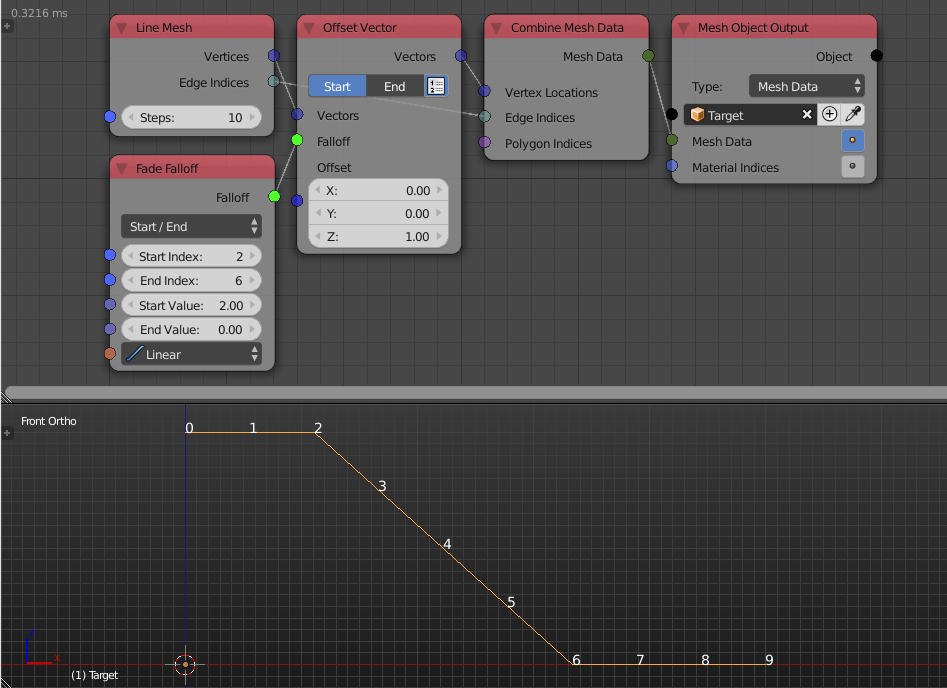## Description

This node creates an index based falloff that associates input Start Value to all objects with index less than some input index and the input End Value to all objects with index more than some input index, while the objects at indices in between are associated with values in between the Start Value and End Value evaluated at the input interpolation.

## IllustrationThe examples above shows the fade falloff node in action. We offset the vertices of the line by one unit in the z-axis and used the Fade Falloff node as a factor for that offset. The Start Index is set to `2` so all vertices with index less than or equal `2` are offset `2` units in the z-axis because the original offset multiplied by the Start Value is `1x2=2`. The End Index is set to `6` so all vertices with index larger than or equal `6` are not offset because the original offset multiplied by the End Value is `1x0=0`. Indices in between however (from `2` to `6`) are offset with amounts linearly changing between `0` and `2`. Had I used a non-linear interpolation, values will no longer linearly change as in the following example:Notice how they are changing exponentially and how the start value affected the offset.

## Options

Options are only different on how the start and end index are defined.

• Start / End - User define start and end indices directly.
• Start / Amount - User define start index and the amount of indices in the interval.
• End / Amount - Use define the end index and the amount of indices in the interval.